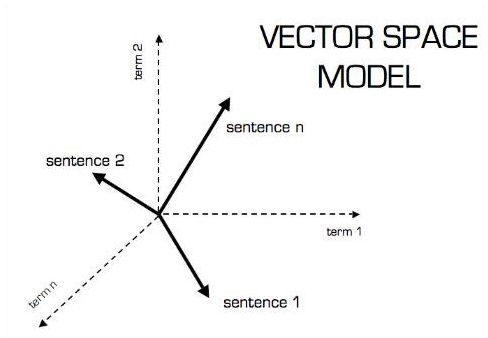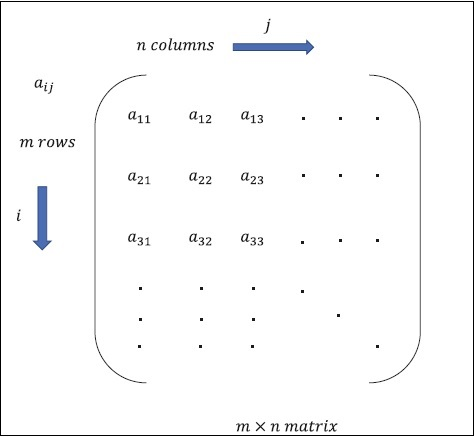# Explain the Vector in TensorFlow?

Explain the Vector in TensorFlow?

by (1.7m points)

## Vector

An array of numbers, which is either continuous or discrete, is defined as a vector. Machine learning algorithms deal with fixed length vectors for better output generation.

Machine learning algorithms deal with multidimensional data so vectors play a crucial role.

The pictorial representation of vector model is as shown below −### Scalar

Scalar can be defined as one-dimensional vector. Scalars are those, which include only magnitude and no direction. With scalars, we are only concerned with the magnitude.

Examples of scalar include weight and height parameters of children.

### Matrix

Matrix can be defined as multi-dimensional arrays, which are arranged in the format of rows and columns. The size of matrix is defined by row length and column length. Following figure shows the representation of any specified matrix.Consider the matrix with “m” rows and “n” columns as mentioned above, the matrix representation will be specified as “m*n matrix” which defined the length of matrix as well.

## Mathematical Computations

In this section, we will learn about the different Mathematical Computations in TensorFlow.

Addition of two or more matrices is possible if the matrices are of the same dimension. The addition implies addition of each element as per the given position.

Consider the following example to understand how addition of matrices works −

Example:A=B=thenA+B=[1+53+72+64+8]=Example:A=B=thenA+B=[1+52+63+74+8]=

### Subtraction of matrices

The subtraction of matrices operates in similar fashion like the addition of two matrices. The user can subtract two matrices provided the dimensions are equal.

Example:ABthenABExample:A−B−thenA−B−[1−52−63−74−8]−[−4−4−4−4]

### Multiplication of matrices

For two matrices A m*n and B p*q to be multipliable, n should be equal to p. The resulting matrix is −

C m*q

A=B=A=B=

c11==1×5+2×7=19c12==1×6+2×8=22c11==1×5+2×7=19c12==1×6+2×8=22

c21=[<span style="f